Wolfgang Trutschnig's homepage

# 1 Summary

The R-package qad (short for quantification of asymmetric dependence) allows to quantify/estimate the (directed) dependence of two variables $$X$$ and $$Y$$. The implemented estimator is copula based, hence scale-invariant, and estimates a directed (population based) dependence measure $$q(X,Y)$$ attaining values in $$[0,1]$$ and having the following main property: $$q(X,Y)$$ is $$1$$ if and only if $$Y$$ is a function of $$X$$ (knowing $$X$$ means knowing $$Y$$) and $$0$$ if and only if $$X$$ and $$Y$$ are independent (no information gain). While the Pearson correlation coefficient assesses only linear and Spearman rank correlation only monotonic relationships, qad is able to detect any kind of association.

# 2 Motivation

All statistics courses mention independence. Loosely speaking, two random variables $$X$$ and $$Y$$ are called independent, if $$X$$ has no influence on $$Y$$ AND (by definition) vice versa.

Example 2.1 (Rolling a dice twice - independence) Let's assume that $$X$$ and $$Y$$ are random variables, whereby
• $$X$$ is the result of rolling a dice the first time and
• $$Y$$ is the result of rolling the same dice a second time.
If we know $$X$$ (the outcome of rolling the dice the first time), does it help to predict $$Y$$? Obviously not. The probabilities/the distribution of $$Y$$ remain(s) unchanged, i.e., we do not gain any knowledge about $$Y$$ if we know $$X$$ (in fact, $$Y$$ is still uniformly distributed on $$\{1,\ldots,6\}$$) and vice versa. In other words: Knowing $$X$$ does not reduce the uncertainty about $$Y$$ and vice versa (try out the R-Code).
R <- 100000
X <- sample(1:6, R, replace = T)
Y <- sample(1:6, R, replace = T)

#Probability that Y = 1
mean(ifelse(Y == 1, 1, 0))

#Probability that Y = 1 if we know X
probs <- rep(0,6)
for(i in 1:6){
probs[i] <- sum(ifelse(Y == 1 & X == i, 1, 0))/sum(X == i)
}
#Probability that Y = 1 if we know the value of X
probs

What is the opposite of independence? Consider the following example:

Example 2.2 (Almost complete dependence) Suppose that $$(x_1,y_1), \ldots, (x_n, y_n)$$ is a sample of size $$n=40$$ from the model $$Y=X^2+\varepsilon$$ with $$\varepsilon \sim \mathcal{N}(0,0.05)$$ (see Figure 2.1):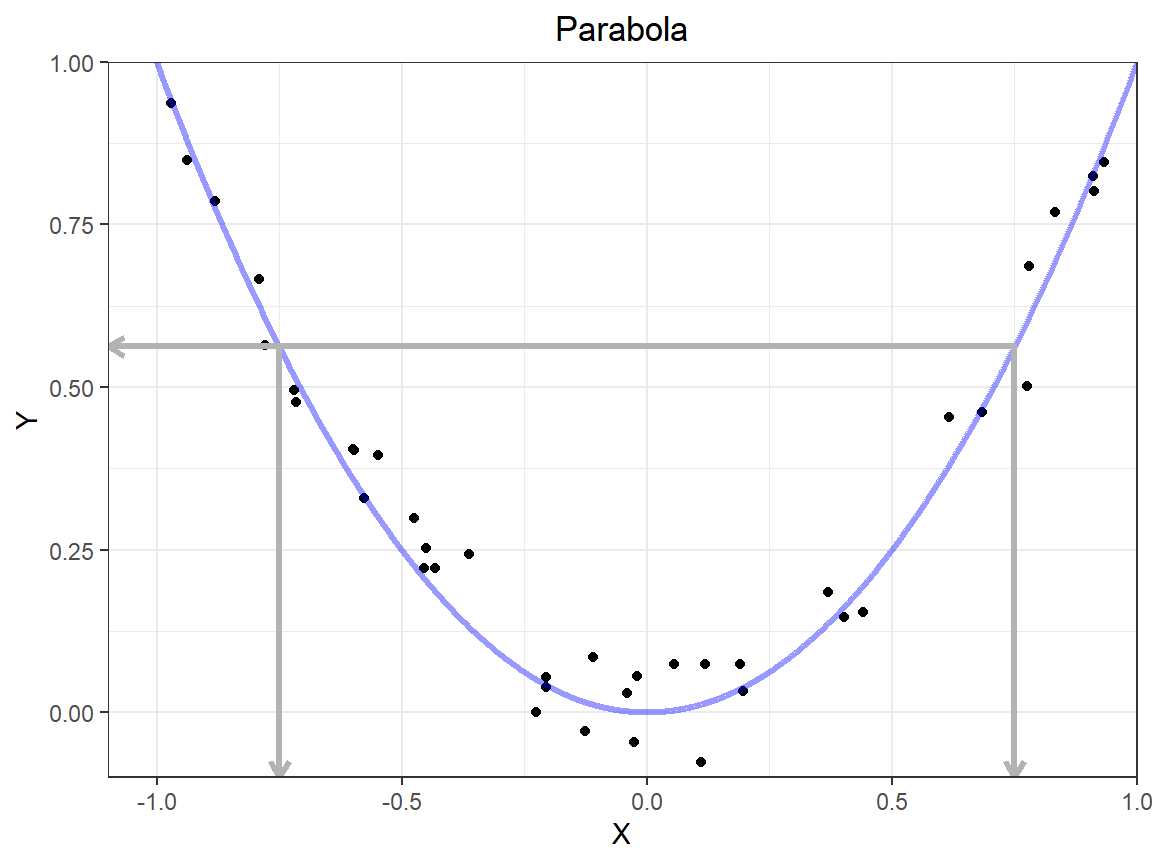Figure 2.1: Sample of size n=40 drawn from the model $$Y=X^2+\varepsilon$$.

Which variable is easier to predict given the value of the other one? Obviously, knowing the value of $$X$$ provides more information about the value of $$Y$$ than vice versa. Indeed, from the (estimated) equation $$Y=X^2$$ we can determine the value of Y, in the other direction, however, we have two possibilities for X given Y.

One natural question arising both theoretically as well as in practise is whether it is possible to quantify and estimate the extent of dependence of two random variables in full generality, i.e., without any distributional assumptions.
Commonly used measures of association such as Pearson $$r$$ or Spearman $$\rho$$ fail to detect any dependence for the situation depicted in Figure 2.1. Furthermore, interchanging $$X$$ and $$Y$$ yields the same result, and we get

• $$r(X,Y)=r(Y,X)=$$ 0.02
• $$\rho(X,Y)=\rho(Y,X)=$$ -0.112

Long story short: methods other than standard correlation are needed.

qad (short for quantification of asymmetric dependence) is a strongly consistent estimator $$q_n(X,Y)$$ of the copula-based, hence scale-invariant directed dependence measure $$q(X,Y)$$ (originally called $$\zeta_1$$) introduced in Trutschnig, 2011. The qad estimator $$q_n(X,Y)$$ was developed and analyzed in Junker, Griessenberger, Trutschnig, 2021 and implemented in the R-package qad in 2020. $$q(X,Y)$$ has the following key properties:

1. $$q(X,Y)$$ can be calculated for all (continuous) random variables $$X$$ and $$Y$$ (without any parametric knowledge about the distribution/model)
2. $$q(X,Y) \in [0,1]$$ (normalization)
3. $$q(X,Y) = 0$$ if and only if $$X$$ and $$Y$$ are independent (independence)
4. $$q(X,Y) = 1$$ if and only if $$Y$$ is a function of $$X$$ (but not necessarily vice versa), i.e., if we have $$Y=f(X)$$, so if we are in the situation that knowing $$X$$ means knowing $$Y$$ exactly (think of Example 2.2 without noise); this situation is usually referred to as complete dependence or full predictability
5. We do not necessarily have $$q(X,Y) = q(Y,X)$$ (asymmetry)
6. Scale changes do not affect $$q(X,Y)$$ (scale-invariance)

Applying qad to the sample in Figure 2.1 yields $$q_n(X,Y)=$$ 0.778, indicating a strong influence of $$X$$ on $$Y$$. On the other hand, $$q_n(Y,X) =$$ 0.439, i.e., the estimated influence of $$Y$$ on $$X$$ is much lower. In other words: $$Y$$ is better predictable by $$X$$ than vice versa, hence the quantity $$a$$ denoting asymmetry in dependence is positive: $$a_n:=q_n(X,Y)-q_n(Y,X)=$$ 0.34.

In what follows the qad approach is sketched and some code examples are presented. We refer to Junker, Griessenberger, Trutschnig, 2021 and Trutschnig, 2011 for a concise mathematical introduction and theoretical results.

# 3 The idea and the approach behind qad

The qad estimator $$q_n(X,Y)$$ is strongly consistent in full generality, i.e., we have $$q_n(X,Y) \approx q(X,Y)$$ for sufficiently large $$n$$ (see Junker, Griessenberger, Trutschnig, 2021). The underlying estimation procedure can be sketched as follows:

• (S0) Suppose that $$(x_1, y_1),\ldots , (x_n, y_n)$$ is a sample from $$(X,Y)$$.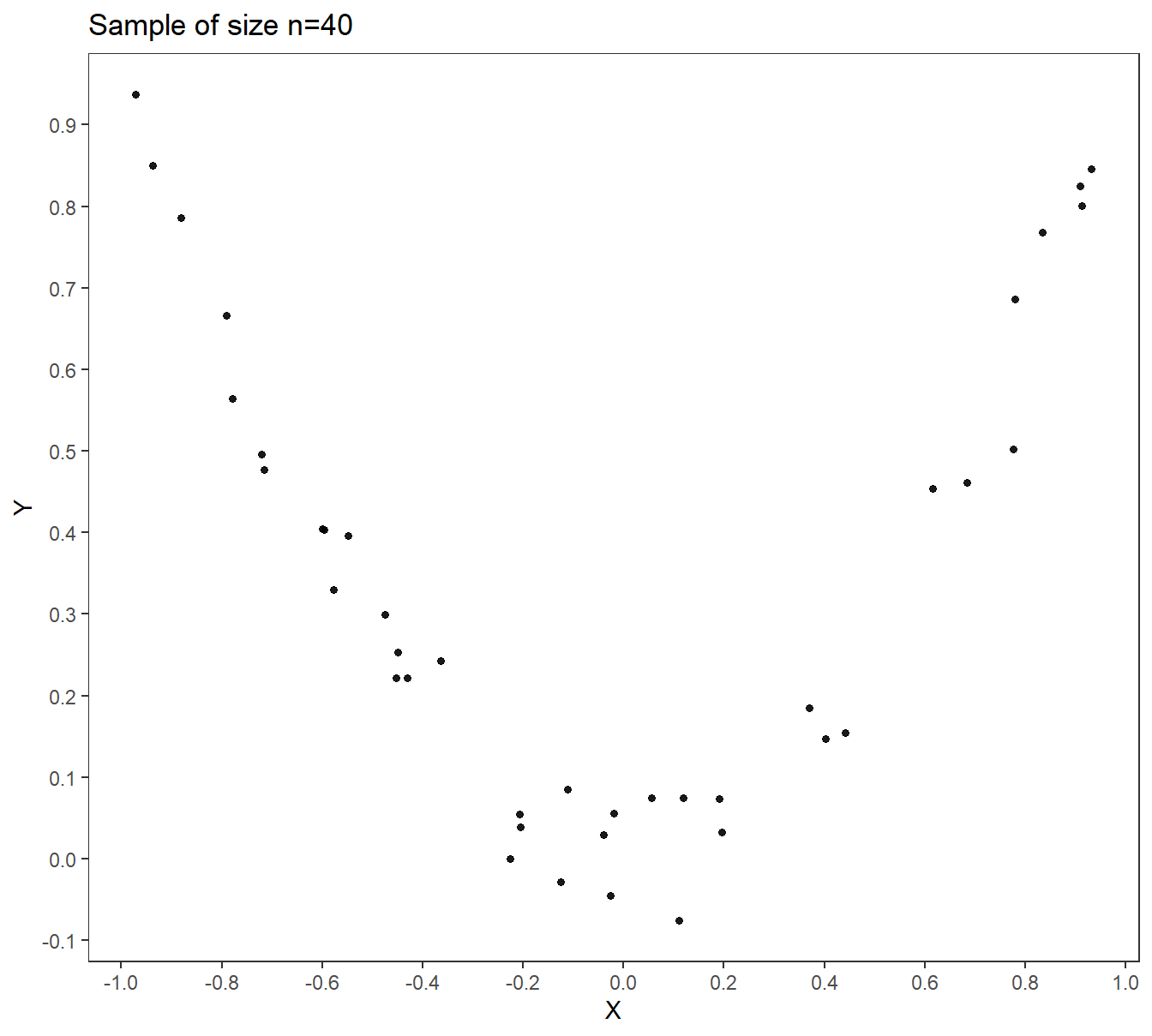Figure 3.1: Sample of size n=40

• (S1) Calculate the normalized ranks of the sample (we get values of the form $$(i/n, j/n)$$ with $$i, j \in \{1, . . . , n\}$$) as well as the so-called empirical copula $$\hat{E}_n$$.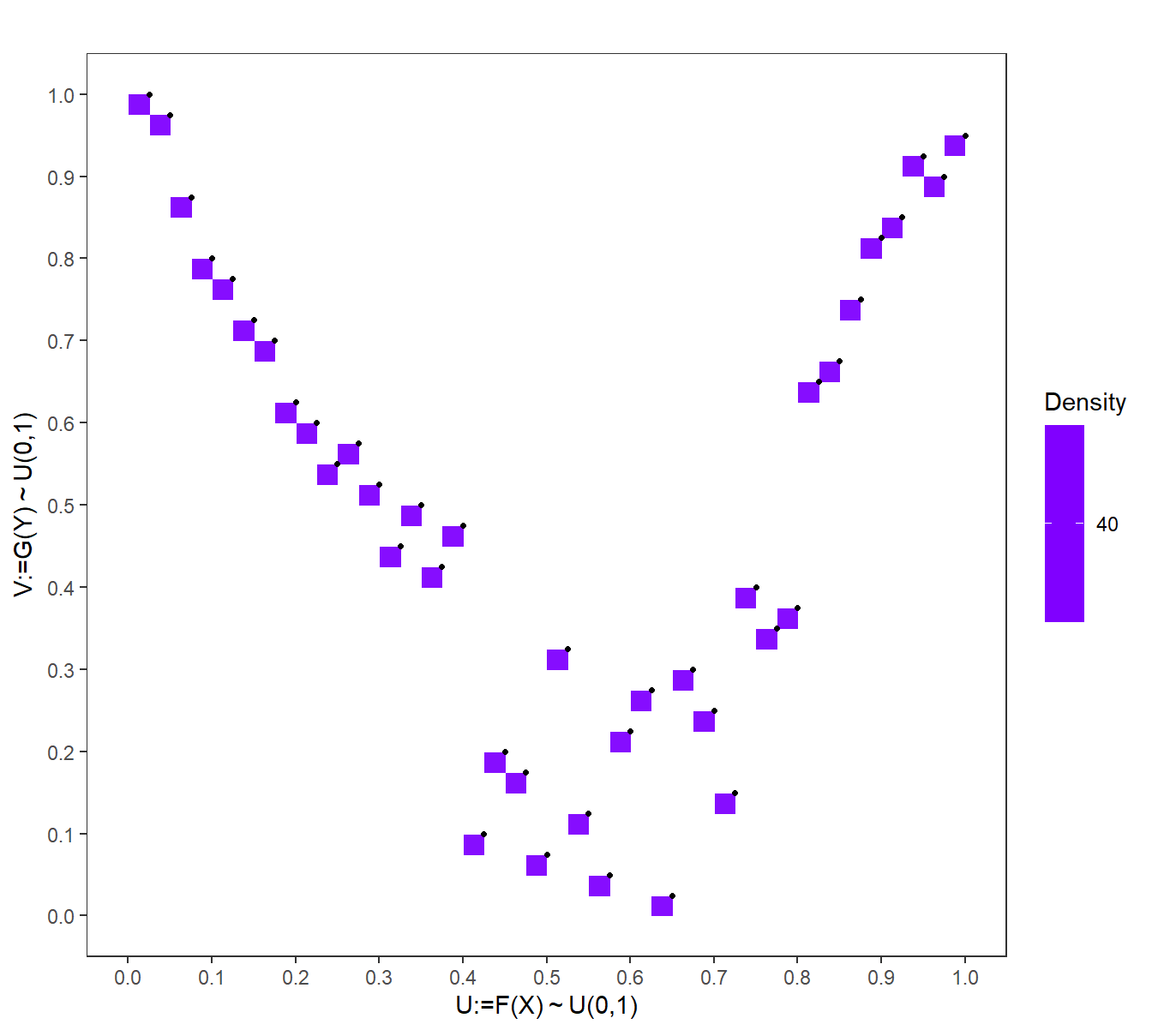Figure 3.2: Empirical copula and normalized ranks (points); notice that the masses are uniform over the squares and that, by construction of the empirical copula, the upper right corner of the squares are the normalized ranks

• (S2) Aggregate the empirical copula to the empirical checkerboard copula $$CB_N(\hat{E}_n)$$ (a.k.a. 2-dimensional histogram in the copula setting). The masses of the little squares are aggregated/summed up to the larger $$N \times N$$ squares; the resolution N depends on the sample size n, in the current case we have $$N=6$$.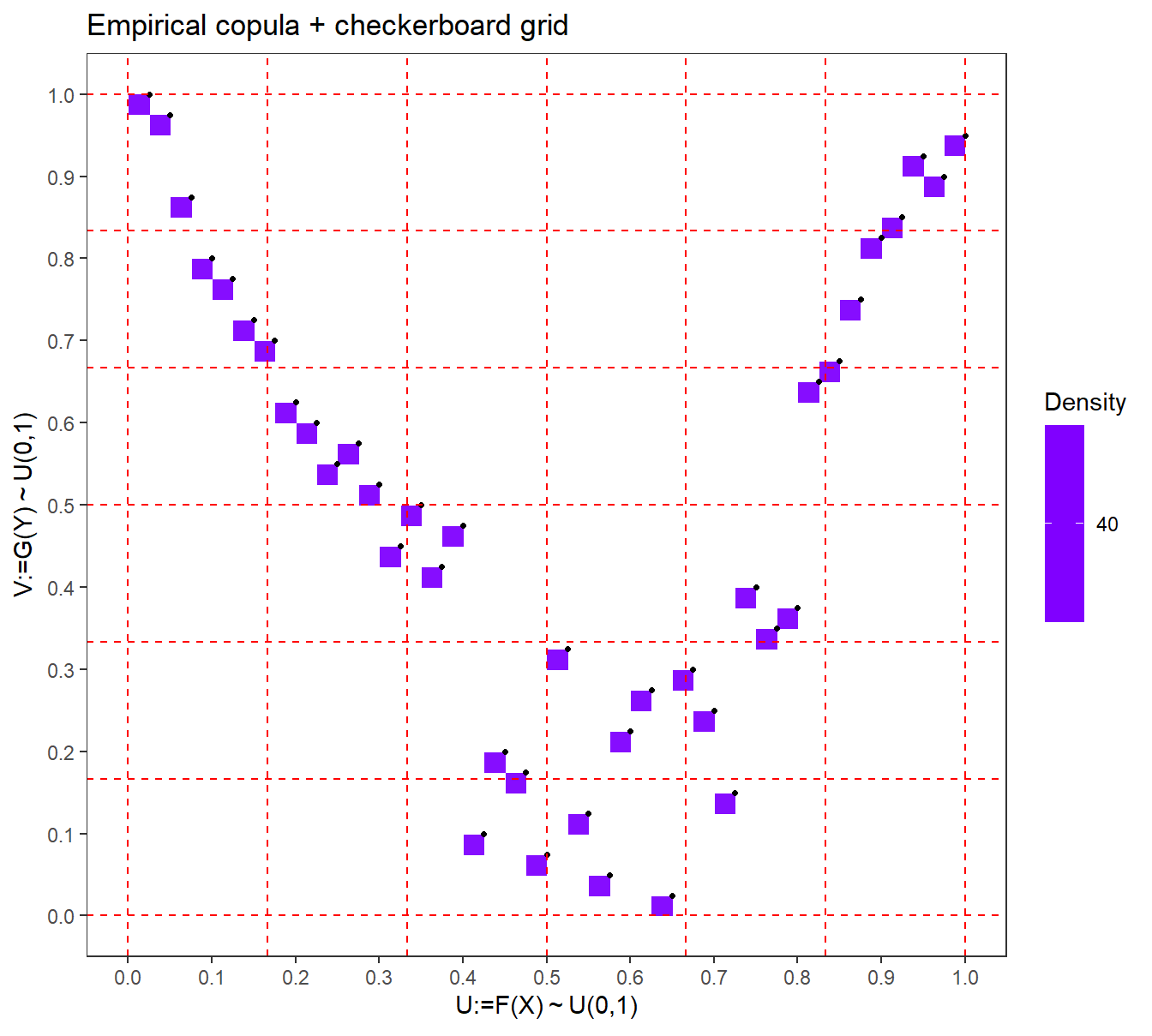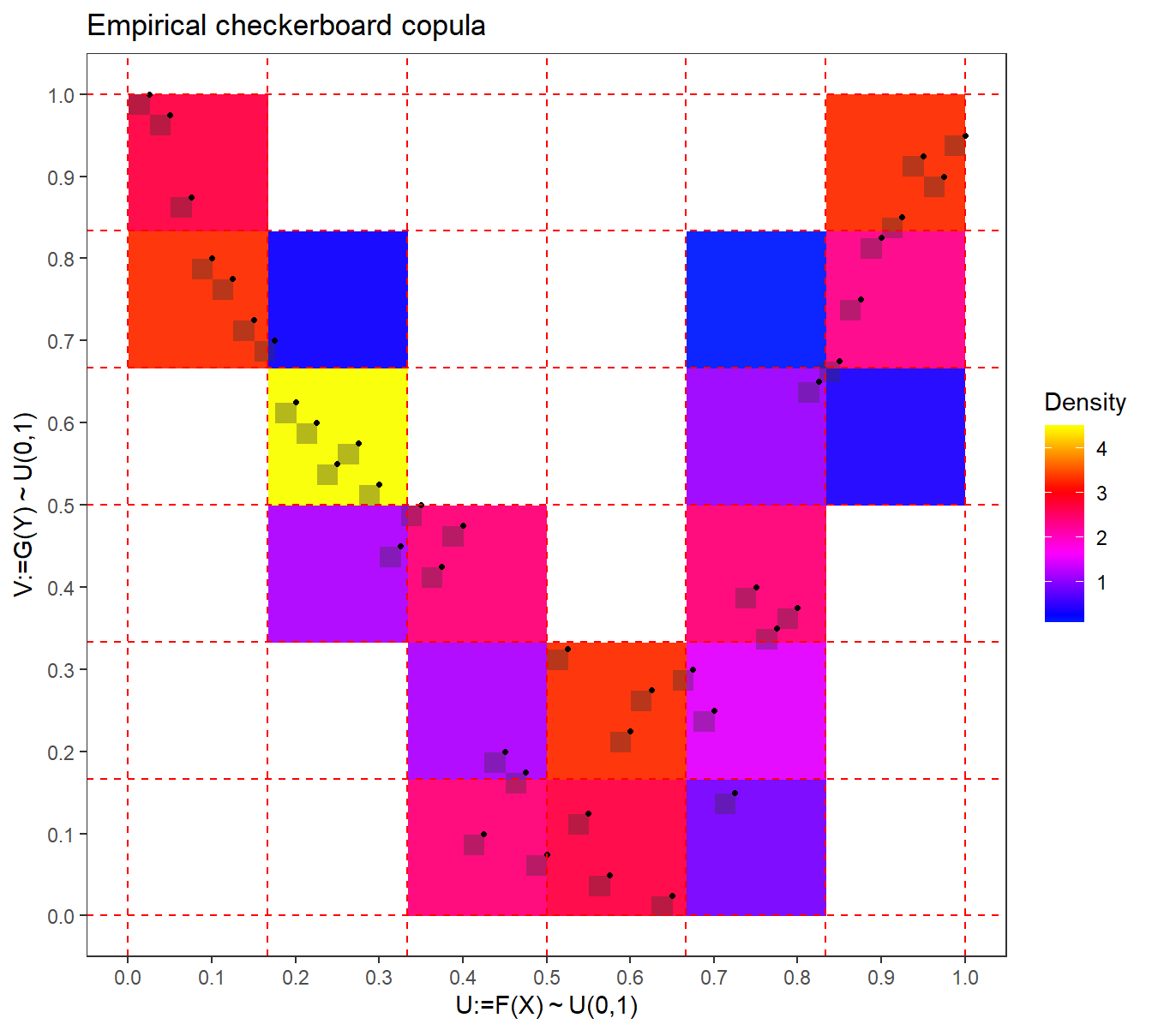Figure 3.3: Empirical copula (left panel) and checkerboard aggregation with resolution $$N=6$$ (right panel)

• (S3) Calculate how different the checkerboard distribution and the uniform distribution on the unit square (modelling independence) are. More precisely, the conditional distribution functions of the checkerboard copula are compared with the distribution function of the uniform distribution on $$[0,1]$$ (in the sense that the area between the graphcs is calculated).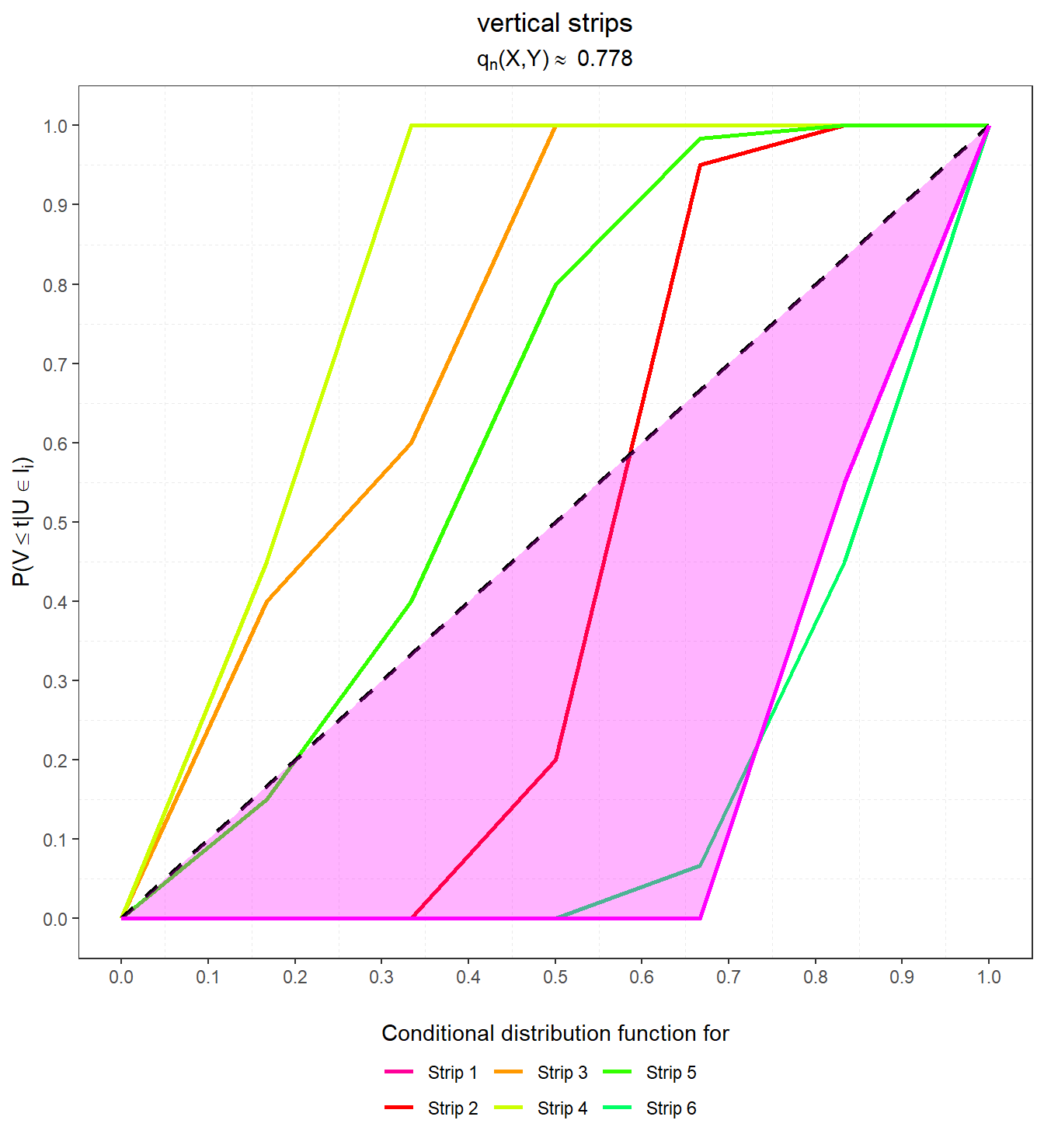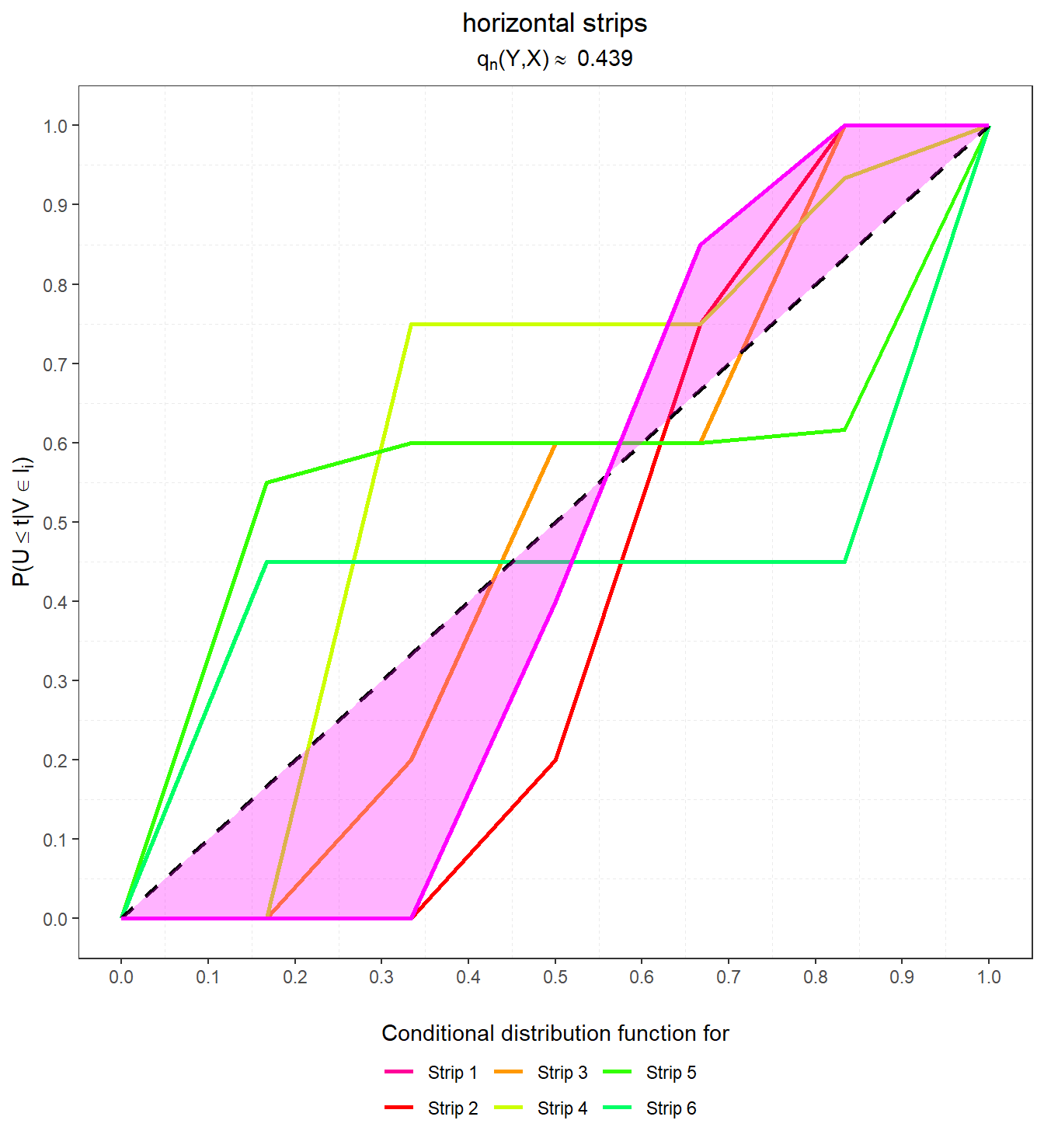Figure 3.4: Distance between the conditional distriubtion functions of the checkerboard copula and the product copula, representing independence, for vertical strips (left panel) and horizontal strips (right panel).

• (S4) Computing the sum of all areas and normalizing appropriately yields $$q_n(X,Y)=$$ 0.778 as well as $$q_n(Y,X) =$$ 0.439.

install.packages("qad")
library(qad)

Some basic instructions for the main functions in qad can be found with

help("qad")
help("qad-package")

# 5 Some examples

## 5.1 Example 1 (Complete dependence):

The following simulated example illustrates again how qad is capable of picking up asymmetry in dependence where standard measures fail. We generate a sample of size $$n=100$$ drawn from a sinusoidal association (a lof of information gain about $$Y$$ by knowing $$X$$, less vice versa) and compute qad in both directions using the function qad().

set.seed(1)

## Step 1: Generate sample
n <- 100
#Underlying Model Y = sin(X) + small.error
X <- runif(n, -10, 10)
Y <- sin(X) + rnorm(n, 0, 0.1)
#Plot the sample
plot(X,Y, pch = 16)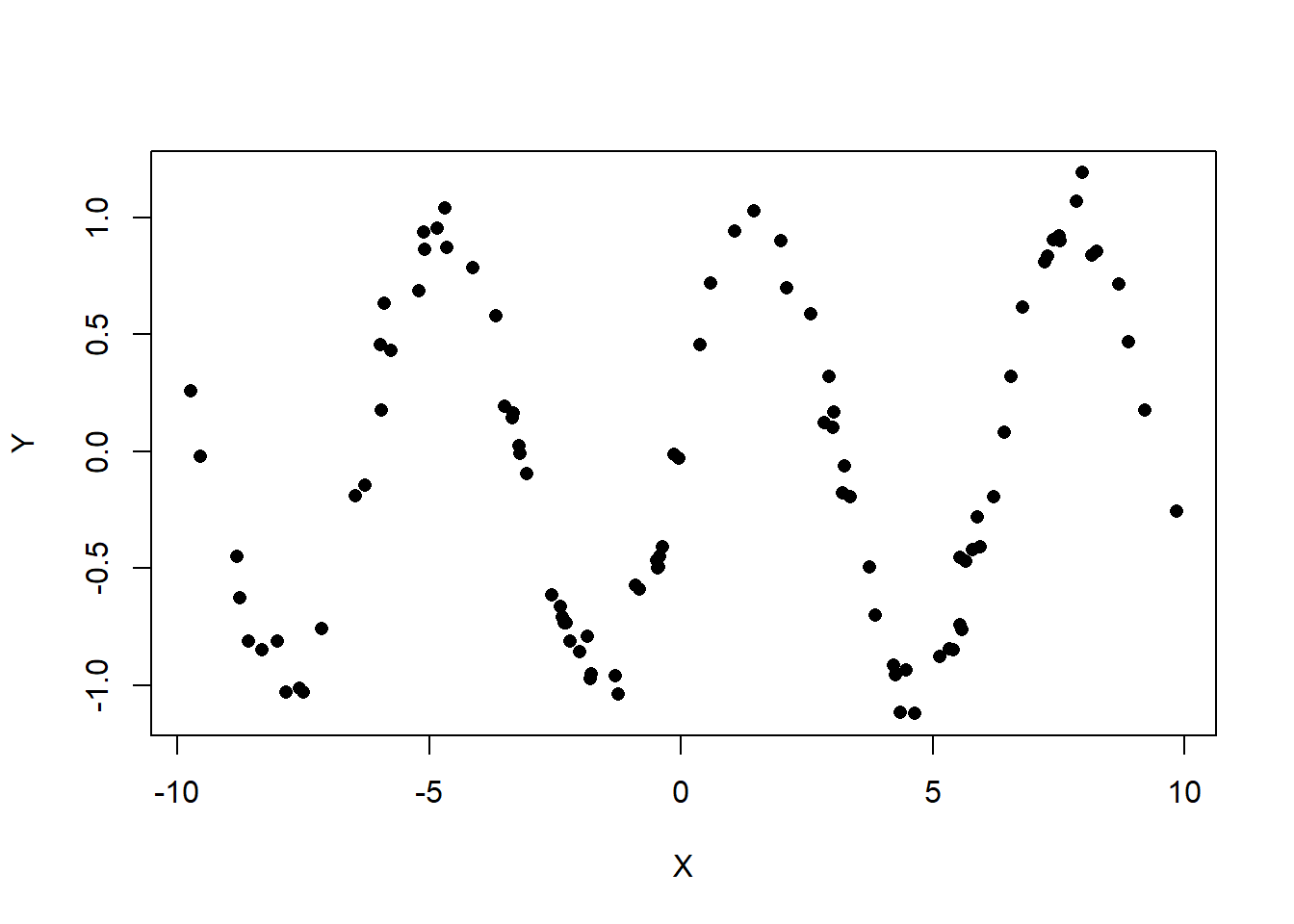#Compute the dependence measure q_n (and the additional p-values obtained by testing for q=0 and a=0)
fit <- qad(X,Y, p.value = T, p.value_asymmetry = T)
#>
#> quantification of asymmetric dependence:
#>
#> Data: x1 := X
#>       x2 := Y
#>
#> Sample Size: 100
#> Number of unique ranks: x1: 100
#>                         x2: 100
#>                    (x1,x2): 100
#> Resolution: 10 x 10
#>
#> Dependence measures:
#>                     q p.values
#>  q(x1,x2)       0.678    0.000
#>  q(x2,x1)       0.309    0.099
#>  max.dependence 0.678    0.000
#>
#>                      a p.values
#>  asymmetry       0.369    0.004

According to $$q(x_1,x_2) > q(x_2,x_1)$$ (in the notation from before meaning that $$q_n(X,Y)>q_n(Y,X)$$) the qad estimator informs us that $$X$$ provides more information about $$Y$$ than vice versa. The qad function additionally calculates the maximum dependence, i.e., $$\max\{q(x_1,x_2),q(x_2,x_1)\}$$, as well as the asymmetry value $$a=q(x_1,x_2)-q(x_2,x_1)$$. Moreover, the output of qad provides p.values obtained by testing for independence and for symmetry in dependence via resampling methods (both hypotheses are rejected at the standard significance level).

## 5.2 Example 2 (Independence):

The following simulated example shows that qad also detects independence. In fact, generating an independent sample of size $$n=100$$ and computing qad in both directions using the function qad() yields the following:

set.seed(1)

## Step 1: Generate sample
n <- 100
#Underlying Model Y = sin(X) + error
X <- rnorm(n, 0, 10)
Y <- rnorm(n, 0, 20)
#Plot the sample
plot(X,Y, pch = 16)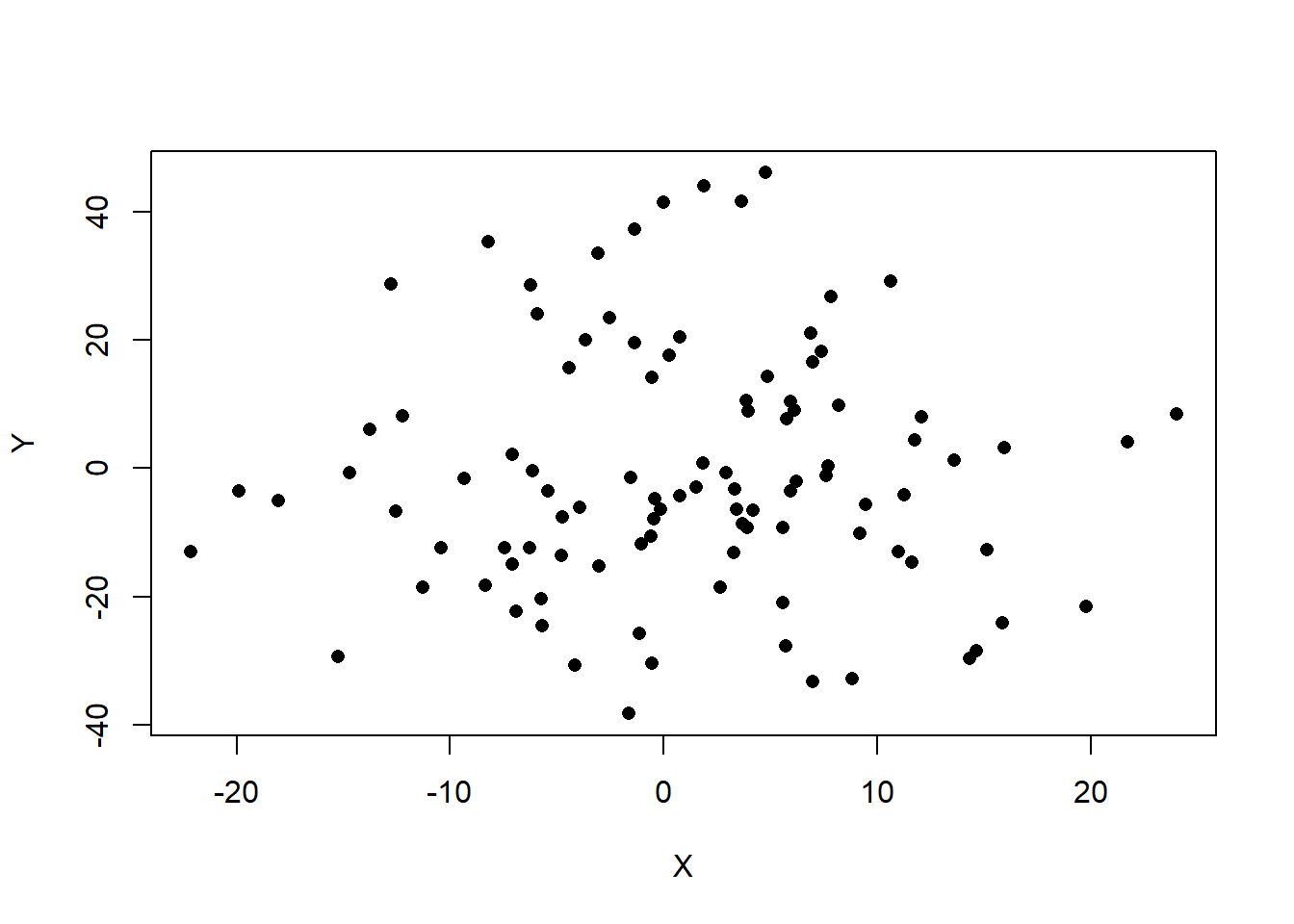#Compute the dependence measure q
fit <- qad(X,Y, p.value = T)
#>
#> quantification of asymmetric dependence:
#>
#> Data: x1 := X
#>       x2 := Y
#>
#> Sample Size: 100
#> Number of unique ranks: x1: 100
#>                         x2: 100
#>                    (x1,x2): 100
#> Resolution: 10 x 10
#>
#> Dependence measures:
#>                     q p.values
#>  q(x1,x2)       0.237    0.697
#>  q(x2,x1)       0.273    0.334
#>  max.dependence 0.273    0.544
#>
#>                       a p.values
#>  asymmetry       -0.037       NA

Although the q-values are greater than $$0$$ (which is always the case by construction - the areas in (S3) can not be smaller than $$0$$), the p.values corresponding to $$q(x_1,x_2)$$ and $$q(x_2,x_1)$$ are 0.697 and 0.334, respectively. Both are much greater than $$0.05$$, so the null hypothesis of independence of the underlying random variables $$X$$ and $$Y$$ is NOT rejected. Notice that for small sample sizes the q-values can be large, nevertheless according to the p.value the null hypothesis of independence is rejected (run the above code with $$n=20$$).

## 6.1 Interpreting the output of qad

The output of qad provides information about the number of unique ranks of the data, the resolution of the checkerboard copula, and the obtained estimates for dependence (in both directions) and asymmetry.

The number of unique ranks is key for calculating the resolution of the checkerboard copula. The larger the sample size, the larger the resolution and the more precise the estimate of the dependence measures (in both directions). When interpreting qad values we recommend to always take into account the resolution. qad vaues corresponding to resolutions of at most 3 should be interpreted cautiously (and by taking into account the small sample size). The qad function prints a warning in such cases:

set.seed(11)
x <- runif(4)
y <- runif(4)
#>
#> quantification of asymmetric dependence:
#>
#> Data: x1 := x
#>       x2 := y
#>
#> Sample Size: 4
#> Number of unique ranks: x1: 4
#>                         x2: 4
#>                    (x1,x2): 4
#> Resolution: 2 x 2
#>
#> Dependence measures:
#>                    q p.values
#>  q(x1,x2)       0.75    0.337
#>  q(x2,x1)       0.75    0.337
#>  max.dependence 0.75    0.337
#>
#>                  a p.values
#>  asymmetry       0       NA
#> Warning in qad.data.frame(X, resolution = resolution, p.value = p.value, :
#> Resolution is less or equal to 3. Results must be interpreted with caution!

As shown above, the main part of the qad output are estimates for the dependence measures $$q(x_1,x_2)$$ (indicating the directed dependence between $$x_1$$ and $$x_2$$, or equivalently, the information gain about $$x_2$$ by knowing $$x_1$$), $$q(x_2,x_1)$$ (indicating the directed dependence between $$x_2$$ and $$x_1$$, or equivalently, the information gain about $$x_1$$ by knowing $$x_2$$), the maximal dependence and the measure of asymmetry (which can be interpreted as estimate for the difference of the predictability of $$x_2$$ given knowledge on $$x_1$$ and the predictability of $$x_1$$ given knowledge on $$x_2$$). By default, in case of no ties in the data, the resolution $$N$$ is chosen as $$\lfloor n^\frac{1}{2} \rfloor$$, in case of ties, the sample size $$n$$ is replaced by the minimum number of unique values of $$x_1$$ and $$x_2$$.

## 6.2 qad as prediction tool

As useful by-product of the calculation of the dependence measure qad, the random variables $$Y$$ given $$X=x$$ (in the sequel denoted by $$Y \vert X=x$$) and $$X$$ given $$Y=y$$ can be predicted for every $$x\in Range(X)$$ and $$y\in Range\left(Y\right)$$ in full generality, i.e., without any prior assumptions on the distribution or the real regression function. Using the afore-mentioned empirical checkerboard copula an estimator for the distribution function of the conditional random variable $$Y|X=x$$ can be derived. This conditional distribution function is then used to return the probability of the event that $$Y|X=x$$ lies in predefined intervals. Thus, contrary to regression and many machine learning algorithms focusing on predicting only one value for $$Y|X=x$$ (usually the estimate for the conditional expectation $$\mathbb{E}(Y|X=x)$$) qad also returns probabilities for $$Y|X=x$$ to be contained in a number of intervals (dependent on the sample size) and thereby provides additional useful information. The subsequent example illustrates the forecasting procedure:

#Generate sample
set.seed(1)
n <- 250
y <- runif(n, -10, 10)
x <- y^2 + rnorm(n, 0, 6)
df <- data.frame(x=x,y=y)

fit <- qad(df, print = FALSE)

#Predict the values of Y given X=0 and Y given X=65)
pred <- predict.qad(fit, values=c(0,65), conditioned=c("x1"), pred_plot = FALSE)
#Output as data.frame
pred$prediction #Output as plot pp <- pred$plot + theme_classic() + theme(plot.title = element_blank(), legend.position = c(0.9,0.5))
#pp
#>    Interval lowerBound upperBound x1=0 x1=65
#> 1        I1 -9.7384485 -7.9002472 0.00  0.18
#> 2        I2 -7.9002472 -6.3766335 0.00  0.06
#> 3        I3 -6.3766335 -5.1040545 0.00  0.00
#> 4        I4 -5.1040545 -3.8711348 0.00  0.00
#> 5        I5 -3.8711348 -2.8654618 0.00  0.00
#> 6        I6 -2.8654618 -1.7375158 0.14  0.00
#> 7        I7 -1.7375158 -0.4729751 0.28  0.00
#> 8        I8 -0.4729751  0.3715227 0.16  0.00
#> 9        I9  0.3715227  1.9618484 0.28  0.00
#> 10      I10  1.9618484  3.0174093 0.08  0.00
#> 11      I11  3.0174093  4.4742189 0.06  0.00
#> 12      I12  4.4742189  5.5782935 0.00  0.00
#> 13      I13  5.5782935  7.2867894 0.00  0.10
#> 14      I14  7.2867894  8.4814894 0.00  0.60
#> 15      I15  8.4814894  9.8536812 0.00  0.06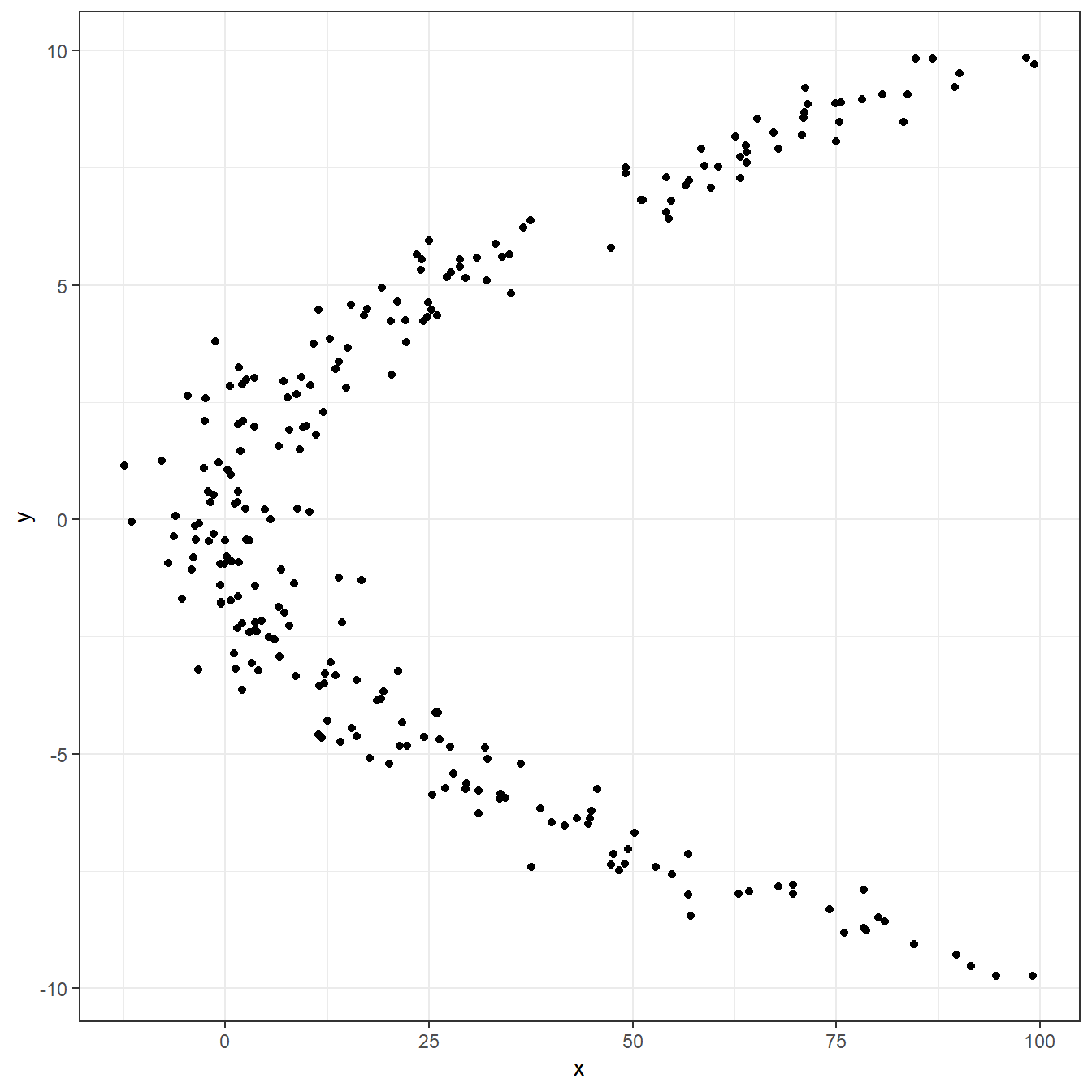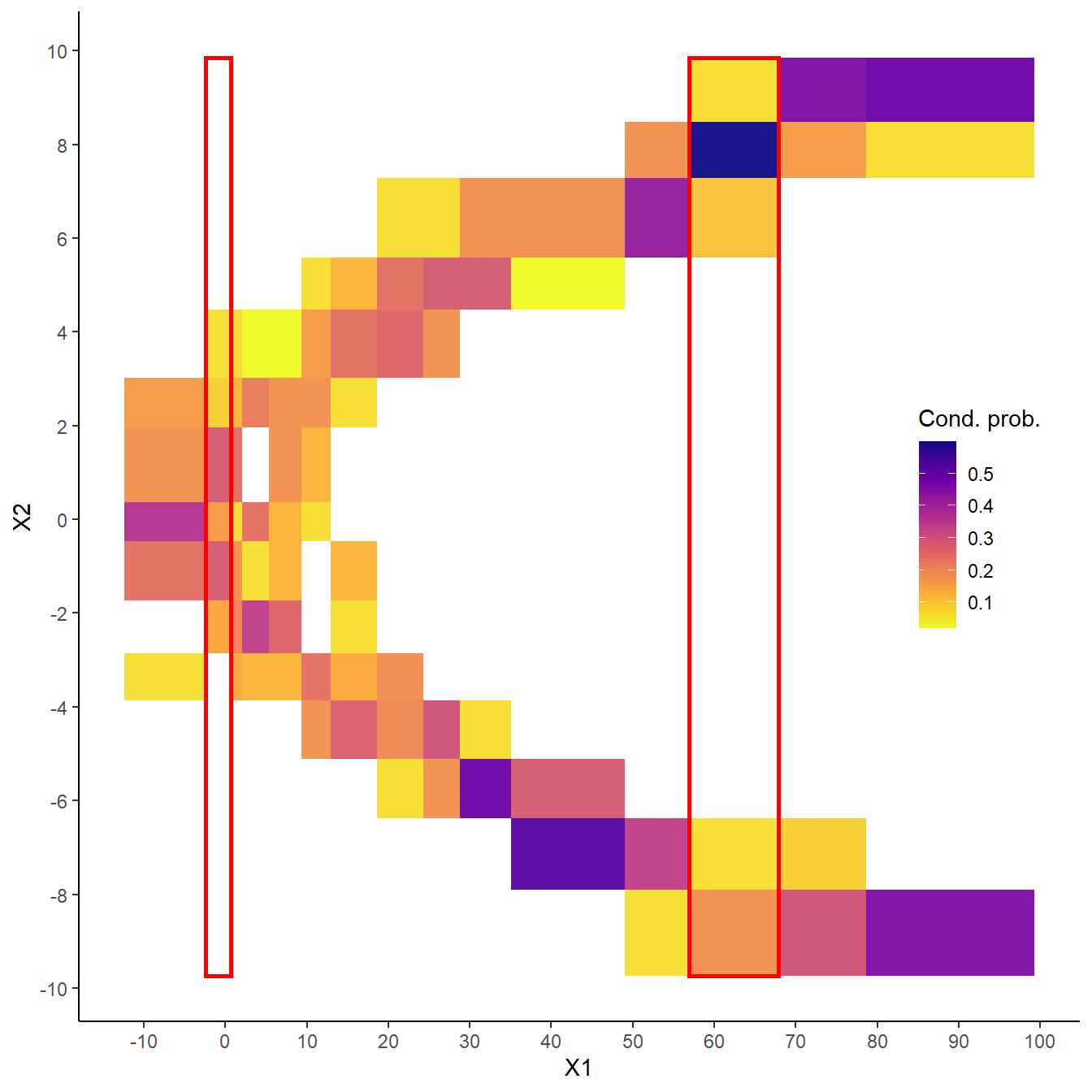Figure 6.1: Sample of the data (left panel) and prediction probabilities of qad (right panel)

The output of predict.qad() consists of the intervals (lower and upper boundary) and corresponding estimated probabilities. For the data in Figure 6.1 we get that given $$X=65$$, the probability for $$Y$$ lying between $$-9.74$$ and $$-6.38$$ is $$0.24$$, and for lying between $$5.58$$ and $$9.85$$ is $$0.76$$.

## 6.3 Data with ties

The qad estimator has originally been developed for data without ties, i.e., for random variables $$X$$ and $$Y$$ having continuous distribution functions. However, numerous simulations have shown that qad also performs well for data with ties (same entries appearing many times). Formally, the qad approach always calculates a checkerboard copula based on the empirical copula, no matter if the data have ties or not. Nevertheless in the setting with ties the empirical copula may build upon rectangles instead of squares and the precision of the estimator may decrease.

Mathematically speaking, suppose that $$(x_1, y_1), \ldots, (x_n, y_n)$$ is a sample of $$(X, Y)$$ and let $$H_n$$ denote the bivariate empirical distribution function and $$F_n$$, $$G_n$$ the univariate empirical marginal distribution functions. Then there exists a unique subcopula $$E_n': Range(F_n) \times Range(G_n) \to Range(H_n)$$ defined by $E_n'(s_1,s_2) = \frac{1}{n} \sum_{i=1}^n 1_{[0,s_1] \times [0,s_2]} (F_n(x_i), G_n(y_i))$ for all $$(s_1, s_2) \in Range(F_n) \times Range(G_n)$$. Extending $$E_n'$$ to full $$[0, 1]^2$$ via bilinear interpolation yields a unique absolutely continuous copula $$E_n$$ which we refer to as empirical copula. For this very copula the checkerboard aggregation and the dependence measure of the latter is calculated. The following example illustrates the approach in case of ties:

set.seed(1)
n <- 30
x <- sample(-10:10, n, replace = T)
y <- x^2
fit <- qad(x,y, print = F)
#> Warning in qad.data.frame(X, resolution = resolution, p.value = p.value, :
#> Resolution is less or equal to 3. Results must be interpreted with caution!
plot(fit, addSample = T, copula = T, density = T, point.size = 1.1)